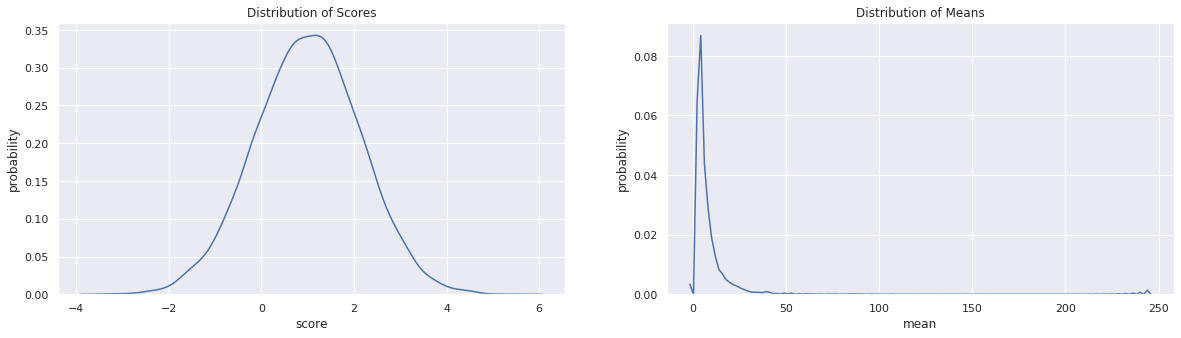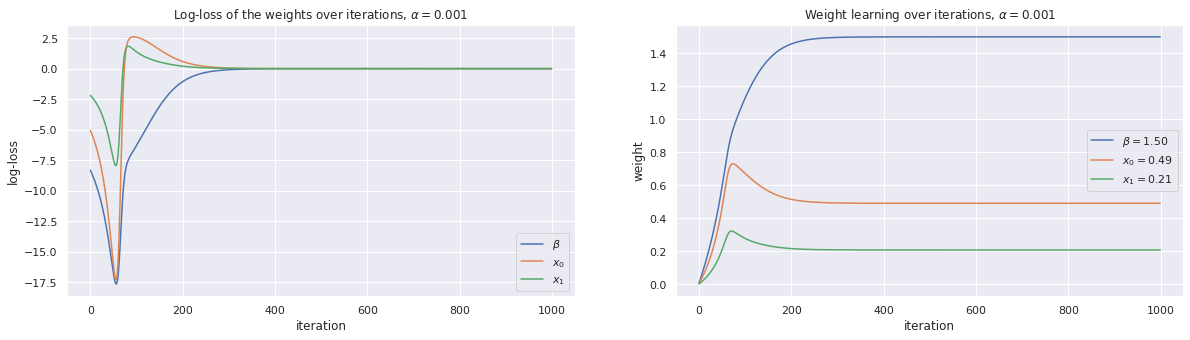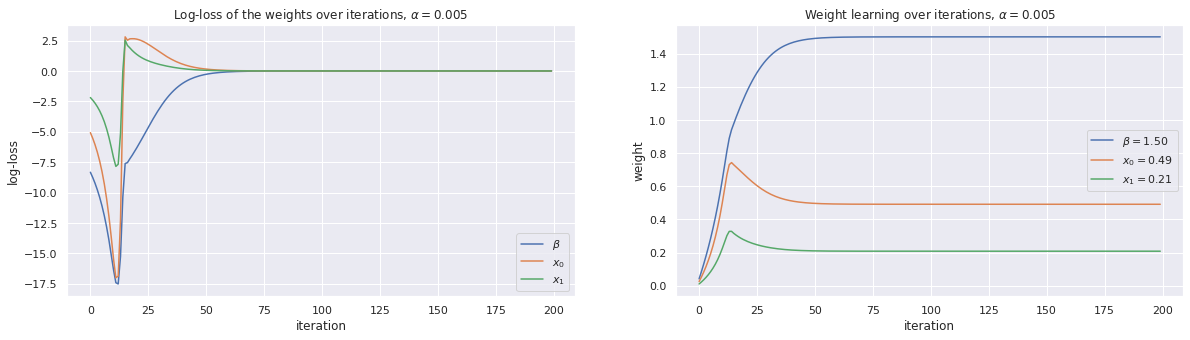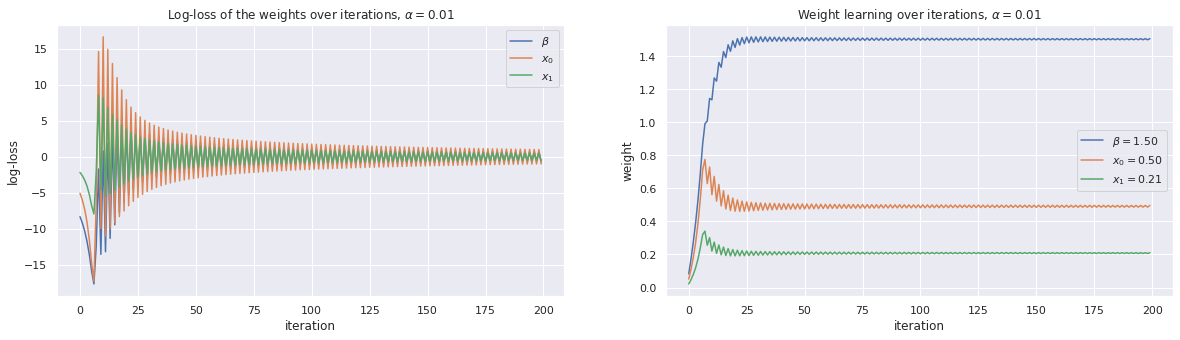# 4. Poisson Regression, Gradient Descent

In this notebook, we will show how to use gradient descent to solve a Poisson regression model. A Poisson regression model takes on the following form.

$$\operatorname{E}(Y\mid\mathbf{x})=e^{\boldsymbol{\theta}' \mathbf{x}}$$

where

• $$x$$ is a vector of input values

• $$\theta$$ is a vector weights (the coefficients)

• $$y$$ is the expected value of the parameter for a Poisson distribution, typically, denoted as $$\lambda$$

Note that Scikit-Learn does not provide a solver a Poisson regression model, but statsmodels does, though examples for the latter is thin.

## 4.1. Simulate data

Now, let’s simulate the data. Note that the coefficients are $$[1, 0.5, 0.2]$$ and that there is error $$\epsilon \sim \mathcal{N}(0, 1)$$ added to the simulated data.

$$y=e^{1 + 0.5x_1 + 0.2x_2 + \epsilon}$$

In this notebook, the score is denoted as $$z$$ and $$z = 1 + 0.5x_1 + 0.2x_2 + \epsilon$$. Additionally, $$y$$ is the mean for a Poisson distribution. The variables $$X_1$$ and $$X_2$$ are independently sampled from their own normal distribution $$\mathcal{N}(0, 1)$$.

After we simulate the data, we will plot the distribution of the scores and means. Note that the expected value of the output $$y$$ is 5.2.

:

%matplotlib inline
import numpy as np
import matplotlib.pyplot as plt
import seaborn as sns
from numpy.random import normal
from scipy.stats import poisson

np.random.seed(37)
sns.set(color_codes=True)

n = 10000
X = np.hstack([
np.array([1 for _ in range(n)]).reshape(n, 1),
normal(0.0, 1.0, n).reshape(n, 1),
normal(0.0, 1.0, n).reshape(n, 1)
])
z = np.dot(X, np.array([1.0, 0.5, 0.2])) + normal(0.0, 1.0, n)
y = np.exp(z)


## 4.2. Visualize data

:

fig, ax = plt.subplots(1, 2, figsize=(20, 5))

sns.kdeplot(z, ax=ax)
ax.set_title(r'Distribution of Scores')
ax.set_xlabel('score')
ax.set_ylabel('probability')

sns.kdeplot(y, ax=ax)
ax.set_title(r'Distribution of Means')
ax.set_xlabel('mean')
ax.set_ylabel('probability')

:

Text(0, 0.5, 'probability')## 4.3. Solve for the Poisson regression model weights

Now we learn the weights of the Poisson regression model using gradient descent. Notice that the loss function of a Poisson regression model is identical to an Ordinary Least Square (OLS) regression model?

$$L(\theta) = \frac{1}{n} (\hat{y} - y)^2$$

We do not have to worry about writing out the gradient of the loss function since we are using Autograd.

:

import autograd.numpy as np
from autograd.numpy import exp, log, sqrt

# define the loss function
def loss(w, X, y):
y_pred = np.exp(np.dot(X, w))
loss = ((y_pred - y) ** 2.0)
return loss.mean(axis=None)

#the magic line that gives you the gradient of the loss function

def learn_weights(X, y, alpha=0.05, max_iter=30000, debug=False):
w = np.array([0.0 for _ in range(X.shape)])

if debug is True:
print('initial weights = {}'.format(w))

loss_trace = []
weight_trace = []

for i in range(max_iter):
w = w - (loss * alpha)
if i % 2000 == 0 and debug is True:
print('{}: loss = {}, weights = {}'.format(i, loss, w))

loss_trace.append(loss)
weight_trace.append(w)

if debug is True:
print('intercept + weights: {}'.format(w))

loss_trace = np.array(loss_trace)
weight_trace = np.array(weight_trace)

return w, loss_trace, weight_trace

def plot_traces(w, loss_trace, weight_trace, alpha):
fig, ax = plt.subplots(1, 2, figsize=(20, 5))

ax.set_title(r'Log-loss of the weights over iterations, $\alpha=${}'.format(alpha))
ax.set_xlabel('iteration')
ax.set_ylabel('log-loss')
ax.plot(loss_trace[:, 0], label=r'$\beta$')
ax.plot(loss_trace[:, 1], label=r'$x_0$')
ax.plot(loss_trace[:, 2], label=r'$x_1$')
ax.legend()

ax.set_title(r'Weight learning over iterations, $\alpha=${}'.format(alpha))
ax.set_xlabel('iteration')
ax.set_ylabel('weight')
ax.plot(weight_trace[:, 0], label=r'$\beta={:.2f}$'.format(w))
ax.plot(weight_trace[:, 1], label=r'$x_0={:.2f}$'.format(w))
ax.plot(weight_trace[:, 2], label=r'$x_1={:.2f}$'.format(w))
ax.legend()


We try learning the coefficients with different learning weights $$\alpha$$. Note the behavior of the traces of the loss and weights for different $$\alpha$$? The loss function was the same one used for OLS regression, but the loss function for Poisson regression is defined differently. Nevertheless, we still get acceptable results.

### 4.3.1. Use gradient descent with $$\alpha=0.001$$

:

alpha = 0.001
w, loss_trace, weight_trace = learn_weights(X, y, alpha=alpha, max_iter=1000)
plot_traces(w, loss_trace, weight_trace, alpha=alpha)
print(w)

[1.50066529 0.49134304 0.20836951]### 4.3.2. Use gradient descent with $$\alpha=0.005$$

:

alpha = 0.005
w, loss_trace, weight_trace = learn_weights(X, y, alpha=alpha, max_iter=200)
plot_traces(w, loss_trace, weight_trace, alpha=alpha)
print(w)

[1.50066529 0.49134304 0.20836951]### 4.3.3. Use gradient descent with $$\alpha=0.01$$

:

alpha = 0.01
w, loss_trace, weight_trace = learn_weights(X, y, alpha=alpha, max_iter=200)
plot_traces(w, loss_trace, weight_trace, alpha=alpha)
print(w)

[1.50393889 0.49616052 0.21077159]